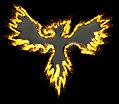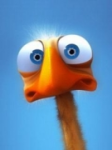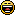###Author Topic: Polygon() Function- Textured, Colored, Rotatable Shapes  (Read 3855 times)

####Hemlos

• To boldy go where no pixel has gone before!
• Global Moderator
• Prof. Inline
••• Posts: 1637
• Particle Hawk##### Polygon() Function- Textured, Colored, Rotatable Shapes
« on: 2009-Dec-14 »
This is something i whipped up today..

You can texture a circular polyvector with color masks for the center and edge of the circle.
It will blend nicely, and you can animate the colors, instead of animating with multiple textures.

Notes:
Really, its a simple function to use, however, I attached a simple button project here also.
You can use a radius collision instead of boxcoll for better accuracy...it is just a quick sample.
To make a circle, you need to set the function to use 36 sides.
If you use the angle input, keep in mind, this rotates the shape, not the image.

Code: (glbasic) [Select]
`FUNCTION DRAWPOLYGON: TextureBMP, X, Y, Radius, EdgeColor, CenterColor, Sides, Angle//Function creates a filled polygon of specified radius// Where:// xy = center of image// radius = width from center, ie you make this the width of image divided by 2, width/2// triangles = # of Sides for polygon// angle = rotate the shape, not the image.  LOCAL Tstep=360.0/Sides FOR Cir = 0 TO 359 STEP Tstep STARTPOLY TextureBMP , 0 CirXS = X + Radius * COS( Cir + Angle ) CirYS = Y + Radius * SIN( Cir + Angle ) CirXE = X + Radius * COS( Cir + Angle - Tstep ) CirYE = Y + Radius * SIN( Cir + Angle - Tstep ) POLYVECTOR CirXS, CirYS, CirXS - X - Radius, CirYS - Y - Radius, EdgeColor POLYVECTOR CirXE, CirYE, CirXE - X - Radius, CirYE - Y - Radius, EdgeColor POLYVECTOR X, Y, -Radius, -Radius, CenterColor ENDPOLY NEXTENDFUNCTION`

Here is the sample button project(screenshot is below the link):

« Last Edit: 2009-Dec-21 by Hemlos »
Volume_of_Earth(km^3) = 4/3*3.14*POW(6371.392896,3)

####Schranz0r

• Prof. Inline
••• Posts: 5064
• O Rly?##### Re: Polygon() Function- Textured, Colored, Rotatable Shapes
« Reply #1 on: 2009-Dec-20 »
cool Function !
IDGArray'sPC:
AMD Ryzen 7 1700 @3.9GHz, 16GB HyperX Fury 3000MHz Ram, ASUS ROG GTX 1060 STRIX 6GB, Windows 10 Pro 64Bit, MSi Tomahawk B350 Mainboard

####Hemlos

• To boldy go where no pixel has gone before!
• Global Moderator
• Prof. Inline
••• Posts: 1637
• Particle Hawk##### Re: Polygon() Function- Textured, Colored, Rotatable Shapes
« Reply #2 on: 2009-Dec-21 »
Thanks F

I optimized the function, it had redundant math in the texuring part:
X-X
Y-Y

Volume_of_Earth(km^3) = 4/3*3.14*POW(6371.392896,3)

####Kitty Hello

• code monkey
••